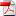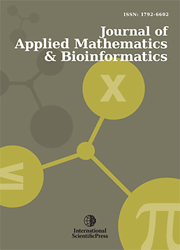# Journal of Applied Mathematics & Bioinformatics

#### Exact traveling wave solutions for an important mathematical physics model

•[ Download ]
• Times downloaded: 1117
• Abstract

In this article, we use the extended tanh function method to obtaine the exact traveling wave solutions involving parameters of nonlinear evolution equation which arises in several physical applications, for example in sound waves in a plasma . When these parameters are taken to be special values, the solitary wave solutions are derived from the exact traveling wave solutions. These studies reveal that the symmetric egularized long-wave equation has a rich variety of solutions.ISSN: 1792-6939 (Online)
1792-6602 (Print)